# Lecture 3 – Strings, Lists, and Arrays¶

## DSC 10, Fall 2023¶

### Announcements¶

• Lab 0 is out and is now due on Saturday, October 7 at 11:59PM.
• It's worthwhile to watch the 🎥 video towards the end on how to navigate DataHub and Jupyter Notebooks.
• Discussion starts today. Your assigned discussion section is based on the lecture section you are enrolled in, unless you were approved to change to a different section. Post privately on Ed with any questions about which section to attend.
• Section A: Wednesday 3-3:50PM in Pepper Canyon Hall 109.
• Section B: Wednesday 4-4:50PM in Pepper Canyon Hall 109.
• Section C: Wednesday 5-5:50PM in Mandeville B-210.
• The first quiz is next week.
• The HDSI Undergraduate Social is tomorrow from 4-6PM on the HDSI Patio.

### Agenda¶

• Data types.
• Strings. 🧶
• Means and medians.
• Lists.
• Arrays.

## Data types¶

### int and float¶

• Every value in Python has a type.
• Use the type function to check a value's type.
• There are two numeric data types:
• int: An integer of any size.
• float: A number with a decimal point.

### int¶

• If you add (+), subtract (-), multiply (*), or exponentiate (**) ints, the result will be another int.
• ints have arbitrary precision in Python, meaning that your calculations will always be exact.

### float¶

• A float is specified using a decimal point.
• A float might be printed using scientific notation.

### The pitfalls of float¶

• floats have limited precision; after arithmetic, the final few decimal places can be wrong in unexpected ways.
• floats have limited size, though the limit is huge.

### Converting between int and float¶

• If you mix ints and floats in an expression, the result will always be a float.
• Note that when you divide two ints, you get a float back.
• A value can be explicity coerced (i.e. converted) using the int and float functions.

## Strings 🧶¶

### Strings 🧶¶

• A string is a snippet of text of any length.
• In Python, strings are enclosed by either single quotes or double quotes.

### String arithmetic¶

When using the + symbol between two strings, the operation is called "concatenation".

### String methods¶

• Associated with strings are special functions, called string methods.
• Access string methods with a . after the string ("dot notation").
• For instance, to use the upper method on string s, we write s.upper().
• Examples include upper, title, and replace, but there are many more.

### Type conversion to and from strings¶

• Any value can be converted to a string using str.
• Some strings can be converted to int and float.

### Concept Check ✅ – Answer at cc.dsc10.com¶

Assume you have run the following statements:

x = 3
y = '4'
z = '5.6'


Choose the expression that will be evaluated without an error.

A. x + y

B. x + int(y + z)

C. str(x) + int(y)

D. str(x) + z

E. All of them have errors

## Means and medians¶

### Describing numerical data¶

• We now know how to store individual numbers (as ints or floats) and pieces of text (as strings). But we often we'll work with sequences, or ordered collections, of several data values.
• For any collection of numbers, say temperatures, it can be helpful to summarize the data by its mean (i.e. average) or median.
• Both mean and median are measures of central tendency – that is, they tell us roughly where the "center" of the data falls.

### The mean (i.e. average)¶

The mean is a one-number summary of a collection of numbers.

For example, the mean of $1$, $4$, $7$, and $12$ is $\frac{1 + 4 + 7 + 12}{4} = 6$.

Observe that the mean:

• Doesn't have to be equal to one of the data points.
• Doesn't have to be an integer, even if all of the data points are integers.
• Is somewhere between the min and max, but not necessarily halfway between.
• Has the same units as the data.

### The median¶

Like the mean, the median is a one-number summary of a collection of numbers.

• To calculate it, sort the data points and pick the number in the middle.
• If there are two middle numbers, we usually pick the number halfway between (i.e. the mean of the middle two).
• Example:
• $\text{Median}(1, 4, 7, 12, 32) = 7$
• $\text{Median}(1, 4, 7, 12) = 5.5$

### Mean vs. median¶

• The mean and median of a dataset can be the same, but they don't need to be. They measure the center of a dataset in two different ways.
• Two different datasets can have the same mean without having the same median, and vice versa.

### Activity¶

1. Find two different datasets that have the same mean and different medians.

2. Find two different datasets that have the same median and different means.

3. Find two different datasets that have the same median and the same mean.

Means and medians are just summaries; they don't tell the whole story about a dataset!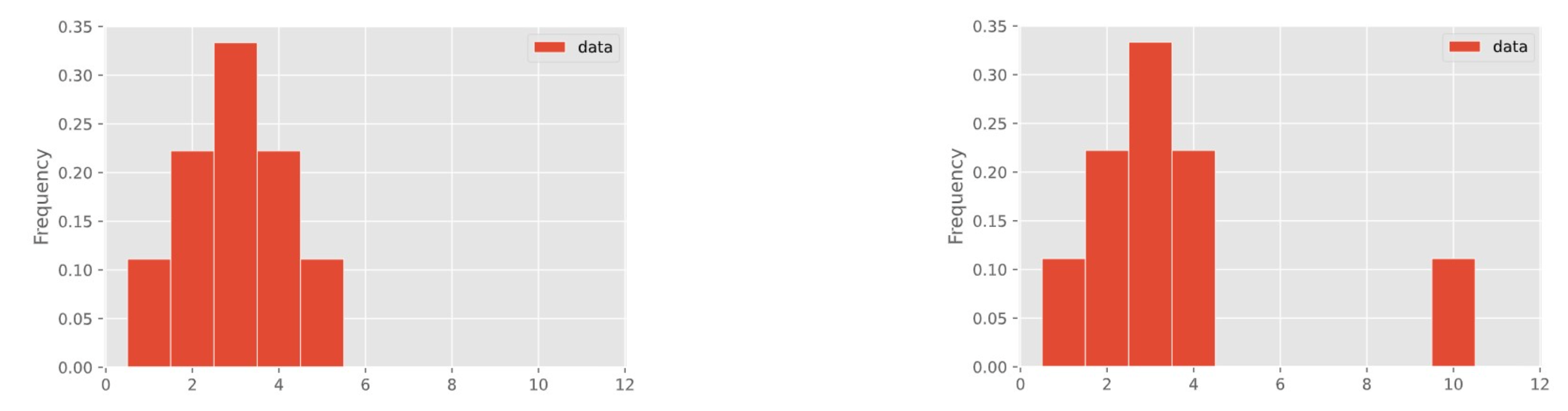In a few weeks, we'll learn about how to visualize the distribution of a collection of numbers using a histogram.

These two distributions have different means but the same median!

## Lists¶

### Average temperature for a week¶

How would we store the temperatures for a week to compute the average temperature?

Our best solution right now is to create a separate variable for each day of the week.

This technically allows us to do things like compute the average temperature:

avg_temperature = 1/7 * (
temp_sunday
+ temp_monday
+ temp_tuesday
+ ...)

Imagine a whole month's data, or a whole year's data. It seems like we need a better solution.

### Lists in Python¶

In Python, a list is used to store multiple values within a single value. To create a new list from scratch, we use [square brackets].

Notice that the elements in a list don't need to be unique!

### Lists make working with sequences easy!¶

To find the average temperature, we just need to divide the sum of the temperatures by the number of temperatures recorded:

### Types¶

The type of a list is... list.

Within a list, you can store elements of different types.

### There's a problem...¶

• Lists are very slow.
• This is not a big deal when there aren't many entries, but it's a big problem when there are millions or billions of entries.

## Arrays¶

### NumPy¶• NumPy (pronounced "num pie") is a Python library (module) that provides support for arrays and operations on them.

• The babypandas library, which you will learn about soon, goes hand-in-hand with NumPy.

• NumPy is used heavily in the real world.
• To use numpy, we need to import it. It's usually imported as np (but doesn't have to be!)

### Arrays¶

Think of NumPy arrays (just "arrays" from now on) as fancy, faster lists.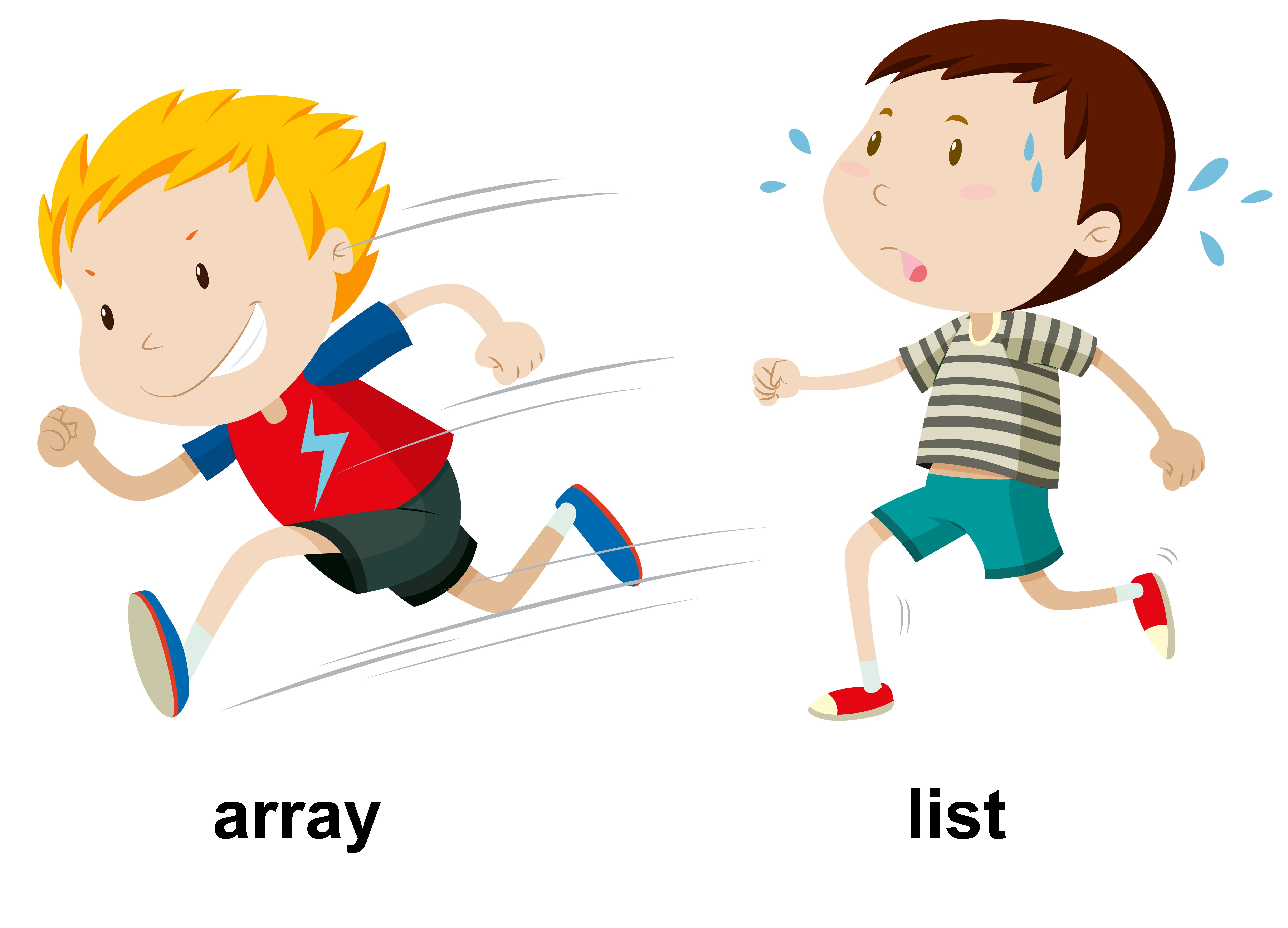To create an array, we pass a list as input to the np.array function.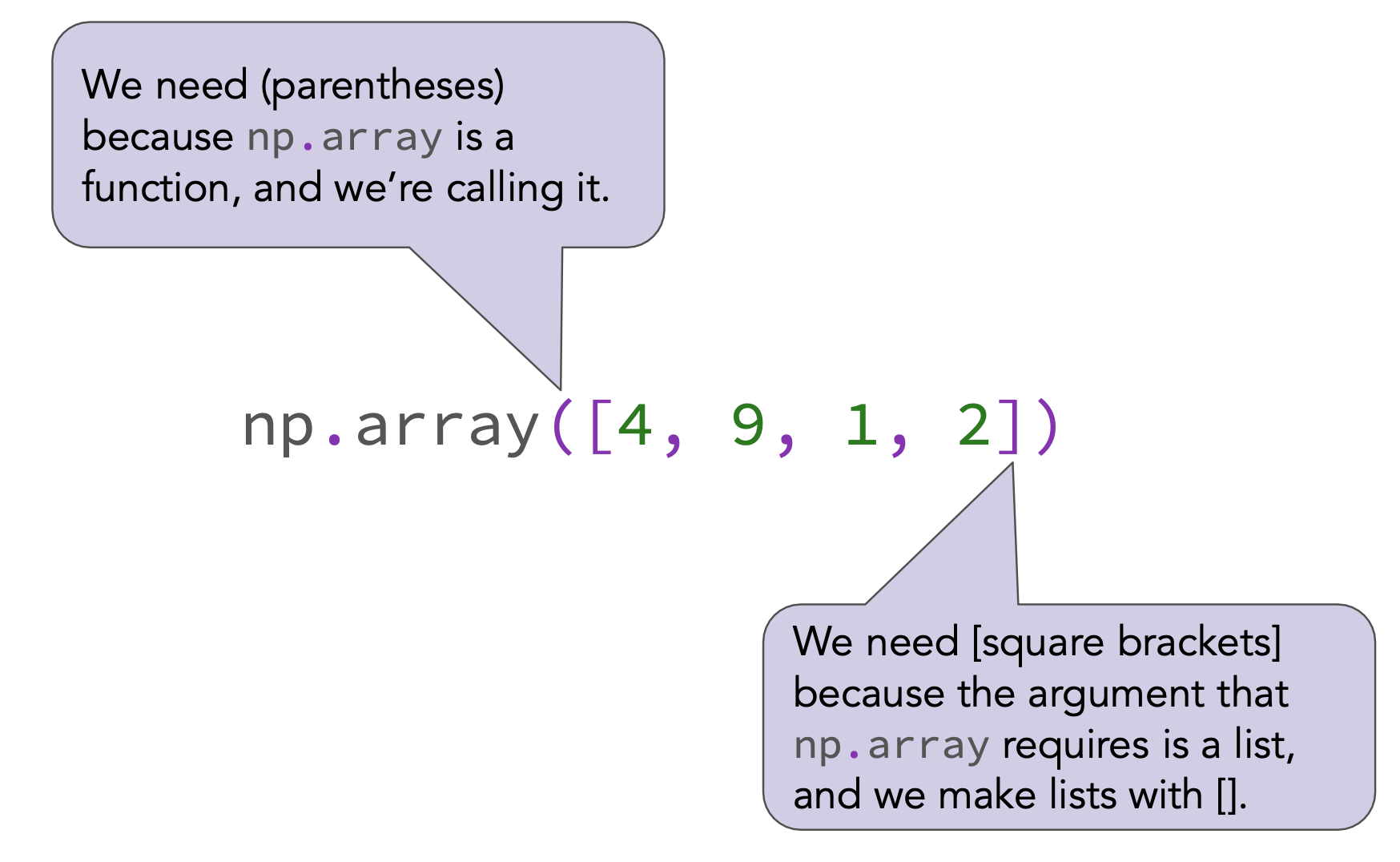### Positions¶

When people wait in line, each person has a position.Similarly, each element of an array (and list) has a position.

### Accessing elements by position¶

• Python, like most programming languages, is "0-indexed."
• This means that the position of the first element in an array is 0, not 1.
• One interpretation is that an element's position represents the number of elements in front of it.
• To access the element in array arr_name at position pos, we use the syntax arr_name[pos].

### Types¶

Earlier in the lecture, we saw that lists can store elements of multiple types.

This is not true of arrays – all elements in an array must be of the same type.

### Array-number arithmetic¶

Arrays make it easy to perform the same operation to every element. This behavior is formally known as "broadcasting".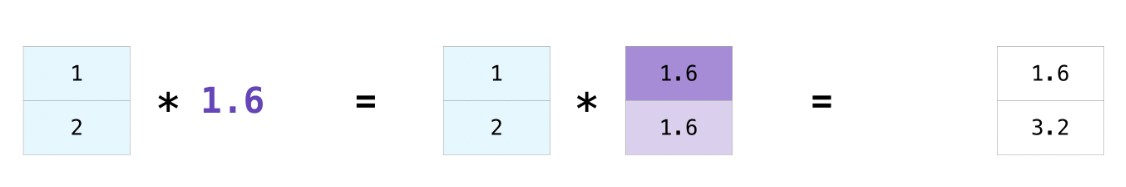Note: In none of the above cells did we actually modify temperature_array! Each of those expressions created a new array.

To actually change temperature_array, we need to reassign it to a new array.

### Element-wise arithmetic¶

• We can apply arithmetic operations to multiple arrays, provided they have the same length.
• The result is computed element-wise, which means that the arithmetic operation is applied to one pair of elements from each array at a time.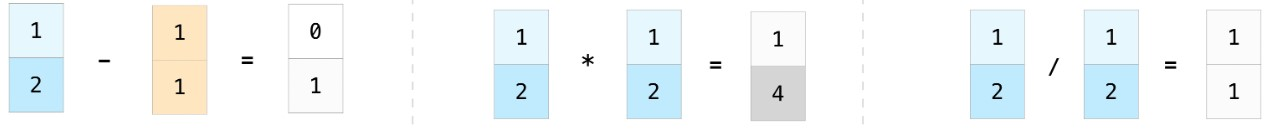## Summary, next time¶

### Summary¶

• Strings are used to store text. Enclose them in single or double quotes.
• Lists and arrays are used to store sequences.
• Arrays are faster and more convenient for numerical operations.
• Access elements by position, starting at position 0.
• Remember to refer to the resources from the start of lecture!

### Next time¶

We'll learn more about arrays and we'll see how to use Python to work with real-world tabular data.